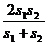# Sample Aptitude Questions of SanDisk

1. The sum of the squares of the digits constituting a two-digit number is 10, and the product of the required number by the number consisting of the same digits written in the reverse order is 403. Find the number.
1. 13
2. 31
3. 41
4. Both 1 & 2
5. None of these

1st condition is satisfied by 1st and 2nd options.
2nd condition is also satisfied by both these options because (1)2 + (3)2 = 10 and (3)2 + (1)2 = 10.
Also 31*13 = 403 and 13*31 = 403. So answer is 4th option.
2. The product of a two-digit number and a number consisting of the same digits written in the reverse order is equal to 2,430. Find the numbers.
1. 45,54
2. 65,56
3. 52,25
4. 35,53
5. None of these

Try by options. 1st is the correct answer because 45*54 = 2430.
1. A garrison of 750 men has provisions for 20 weeks. If at the end of 4 weeks, they are re-inforced by 450 men, how long will the provision last?
1. 8 weeks
2. 12 weeks
3. 14 weeks
4. 15 weeks
5. 10 weeks

750*20 = 750*4+1200*W =>W = 10 weeks
2. If 8 men can reap 80 hectares in 24 days, how many hectares can 36 men reap the same field in 36 days?
1. 540 hectares
2. 450 hectares
3. 420 hectares
4. 489 hectares
5. None of these

H = 80*36/8*36/24= 540 hectares
3. A person covers half of his journey at 30 km/hr and the remaining half at 20 km/hr. The average speed for the whole journey is
1. 18 km/hr
2. 28 km/hr
3. 32 km/hr
4. 20 km/ hr
5. 24 km/hr

Here, s1 = 30 and s2 = 20.therefore Average speed ==2*30*20/30+20 = 24 km/hr.
4. Rajesh covers a certain distance by bus at 16 km/hr and return back at the starting point on a cycle at 9 km/hr. His average speed for the whole journey is
1. 13.54 km/hr
2. 11 km/hr
3. 15.52 km/hr
4. 11.52 km/hr
5. None of these

Here, s1 = 16 and s2 = 9.therefore Average speed == 2*16*9/16*9= 11.52 km/hr.
5. 430% of 25 + 75% of 430 = ?
1. 430
2. 860
3. 516
4. 86
5. 630

430% of 25 + 75% of 430 = x
430/100 ( 25 + 75 ) => x = 430
6. Approximate value of Square root of 2412 – 1592= ?
1. 320
2. 75
3. 150
4. 180
5. 210

2412 – 1592=(241 – 159)(241 + 159) = 82 × 400 = 32800
Square root of 32800 = 180 approximately
7. (364 + 514 - ?)/4=200
1. 162
2. 82
3. 78
4. 68
5. 72